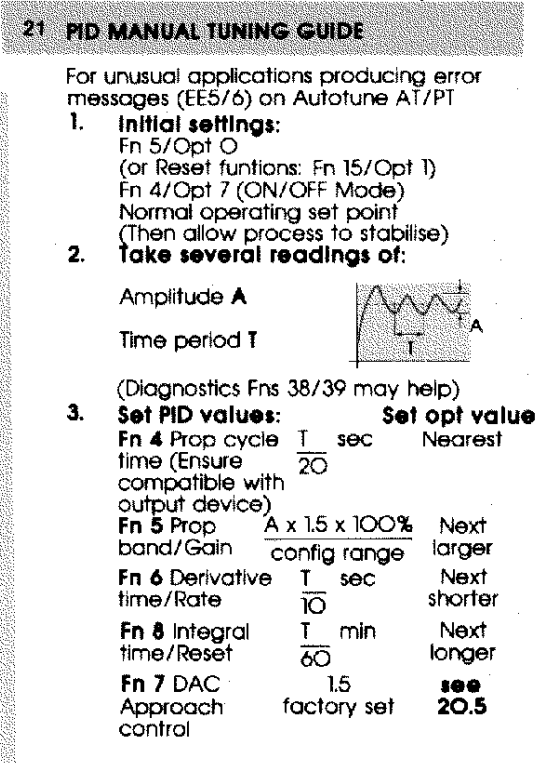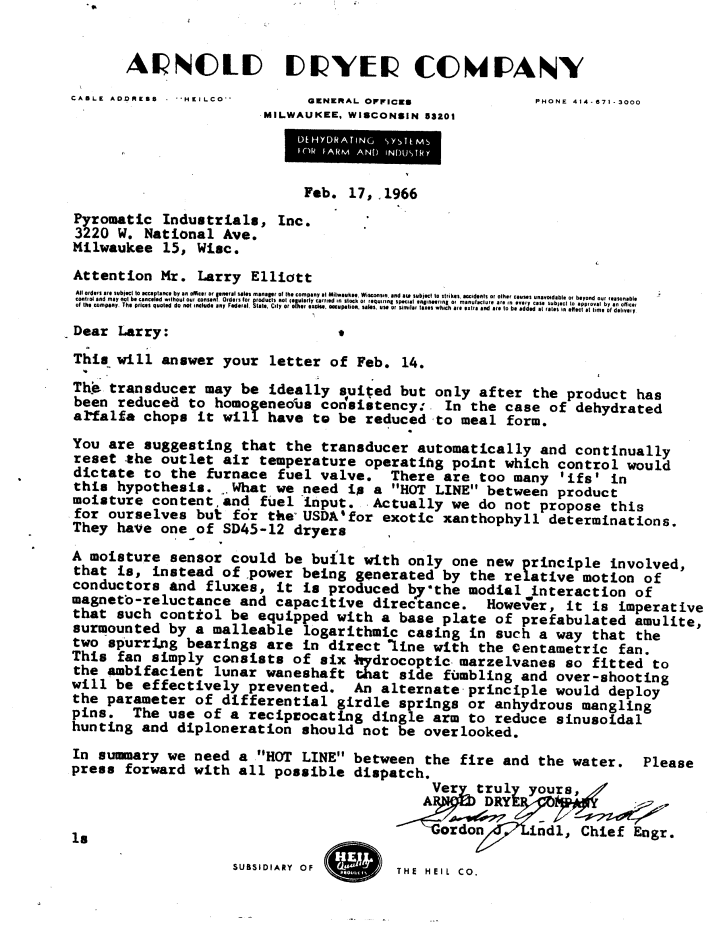•
•Manual PID Tuning Explained

When tuning a PID loop manually, the technique at the left has worked most often for me.  There are a couple confusing things that hopefully can be simplified here.  Follow these steps:

1. Step one is saying put the control in ON/OFF mode.  This means disabling the "I" (integral) and the "D" (derivative) and making the "P" (proportional) very narrow; like 1 degree or less.  For many PID controls this translates to: P=0  I=0 and D=0.  If you are unable to turn off the integral,  it should be set for a very long time so it isn't constantly trying to "reset" the proportional band.  Once these settings are put in, set your temperature and watch the process oscillate around setpoint.  It will go above setpoint and below similar to your kitchen oven at home.  If graphed, it will look like the chart to the left in step 2.  You will need a stopwatch to time the T (period).  I tend to start and stop my stopwatch when the temperature is crossing the setpoint on the way up since it is moving fastest and is easiest to differentiate when to press the stopwatch button.

2.  Calculate the A and the T.  If it goes 40 degrees above setpoint and 30 degrees below, your A=70.  If it takes 2 minutes and 42 seconds to complete one sinusoid (started when temp crosses SP going up...stopped the second time it crosses SP on its way up) then your T= 162 seconds.

3. Set your P = to A x 1.5  (in our example 70 x 1.5 = 105degrees)
Set your I = T or the next larger value (our example 162 seconds)
Set your D = T/10 or the next shorter (our example 16.2 seconds)
Set your Cycle Time if not using 4-20mA (using Time Proportioned output)  Cycle Time = T/20 (our example 8.1 seconds) Side Note: Cylce Time is the time base for the output.  Example: Control is asking for 25% output means the relay will be ON for 8.2seconds x .25 = 2.05 seconds and then it will be OFF for 8.2 seconds x .75 = 6.15 seconds.